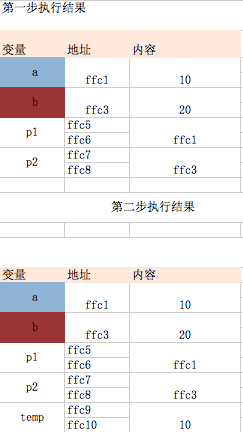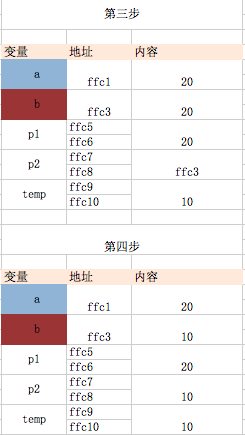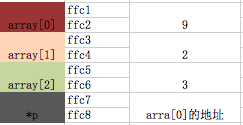# c语言与指针

 16位编译器 32位编译器 64位编译器 Void*(指针变量) 2 4 8

 char a1; int *s=&a1; //会认为a1是int类型所以会取2位(在十六位编译器环境下)

 char a; char *b=&a;//此处并不是赋值操作而是定义了一个指针变量b，然后给b赋值等同于下面     //char *b; //*b声明指针变量    // b=&a; printf("%d",*b); //*b 取出变量a存储的值，也就是访问a的地址

 char *t; *t='1'; //没有指向任何变量的时候，是随机指向，是错误做法 t=100;  //指针变量是用来存储变量地址的，不能随便存储一个常数，除非这个常数刚好等于某个变量的地址

void toplay(char *p1,char  *p2){
char temp=*p1; //第二步
*p1=*p2; //第三步
*p2=temp; //第四步
}
int main(int argc, const char * argv[])
{
//第一步
char a=10;
char b=20;
toplay(&a,&b);

printf("%i",a);
return 0;
}int sumAndMin(int a,int b,int *p){     *p=a-b;     return a+b; } int main(int argc, const char * argv[]) {     int a=10;     int b=20;     int min;     sumAndMin(a, b, &min);     printf("%i",min);     return 0; }

 //指针指向数组元素     int array={9,2,3};     int *p=&array; //int *p=array; 因为array就是array的地址     //改变首个元素的值     *p=10;         //利用指针遍历数组     int *k=&array; //指向的并不是数组，而是数组的第一个元素     for (int i=0; i<3; i++) {         printf("array[%d]=%d\n",i,*(p+i)); // p+i取的是第i个元素的地址 p的值一直都没有改变过     }         for (int i=0; i<3; i++) {         printf("array[%d]=%d\n",i,*(p++)); //  p的值一直在改变，每次遍历都会改变     }         for (int i=0; i<3; i++) {         printf("array[%d]=%d\n",i,*(array+i)); //因为array是个常量所以不能写成array++     }         for (int i=0; pvoid arrayMethod(char s[]){     s=12; } 程序调用 char a[]={1,2}; char *p=a;     arrayMethod(p);     arrayMethod(a);

 void pointMethod(char *p){ p=12; p=14;//修改数组第一个元素的值 p[i]代表第i个元素 } 程序调用： char a[]={1,2};    char *p=a; //arrayMethod(p); //arrayMethod(a); pointMethod(p); pointMethod(a);

1.传统遍历方法

 //遍历一个字符串 void forString(){     char str[]="upsbus.com";     for (int i=0; str[i]!='\0';          i++) {         printf("%c\n",str[i]);     } }

 //通过指针遍历一个字符串 void forStringByPonit(){   //定义的是一个字符串变量     char str[]="upsbus.com";     char *p=str; //*p=&str 因为数组名代表的是数组地址，而数组的地址又是数组第一个元素的地址，所以&可以省略     for (; *p!='\0';          p++) {         printf("%c\n",*p);     } }

 void forStringByPonit2(){ //定义的是一个字符串常量 char *p="upsbus.com"; //这里*p并不是指向整个字符串而是指向第一个字符，因为这是一个指向字符的指针 //char *p; // p="upsbus.com";  拆分过程 printf("%c\n",*p);//打印出来的是第一个元素的内容     for (; *p!='\0';          p++) {         printf("%c\n",*p);     } }

 //字符串常量     char *p1="upsbus.com";     //字符串变量     char p2[]="upsbus.com";

C语言字符串陷阱，以下都是错误代码

 //错误原因是temp是一个常量，代表数组地址即第一个元素的地址，我们不能对一个常量进行赋值     char temp;     temp="upsbus.com";     错误提示：Array type 'char ' is not assignable         char *temp="upsbus.com";     temp=“baidu.com”;//因为temp是一个常量，所以不能对temp进行赋值     temp='a';//此处虽然temp代表的是第一个元素u，看上去可以赋值一个字符，但是temp是一个常量所以不能进行赋值

 //返回函数的指针 char * returnPoint(){     return "coolJune"; }

 int sumNum(int a,int b){     return  a+b; } 程序调用： int (*p)(int ,int ); //int(*p)(int a,int b);       p=sumNum; //方法的地址直接用方法名取        //程序调用     int i=p(2,3);

 //传入一个函数指针，由传入函数的指针决定做什么运算 int calc(int a,int b,int (*p)(int,int)){     return p(a,b); } int (*p)(int ,int );   p=sumNum; int (*s)(int,int); s=minNum; int i=calc(5, 15, s);

08-272695
03-1817
06-091万+
01-1747
10-301万+
03-255349
08-314354
09-27557
10-292908
08-144万+
10-15249
09-253844
12-103737
06-223万+
10-151682
11-0815万+
05-113万+
06-13361
10-241万+
04-23200

### “相关推荐”对你有帮助么？

•非常没帮助
•没帮助
•一般
•有帮助
•非常有帮助被折叠的  条评论 为什么被折叠?到【灌水乐园】发言¥2 ¥4 ¥6 ¥10 ¥20余额支付 (余额：-- )扫码支付获取中扫码支付点击重新获取扫码支付1.余额是钱包充值的虚拟货币，按照1:1的比例进行支付金额的抵扣。
2.余额无法直接购买下载，可以购买VIP、C币套餐、付费专栏及课程。余额充值